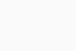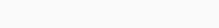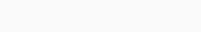# Sorting Associative Array in Python

In this tutorial, we will learn how to sort an associative array in Python.

But let’s first understand what associative array is.
An Associative array contains data in the form of key-value pairs. Each key has a value assigned to it.

In Python, associative arrays are implemented using a dictionary.
For example-

```mydict={}
mydict['s']=2
mydict['a']=5
mydict['z']=1```

Here s, a, z are keys and 2, 5, 1 are their values respectively.

Now, let’s see how to sort this dictionary.

## How to sort Associative Array in Python

We will sort the dictionary in four ways-

1. In ascending order according to key
2. In descending order according to key
3. In ascending order according to value
4. In descending order according to value

## Sorting in ascending alphabetical order according to key

```mydict={}
mydict['s']=2
mydict['a']=5
mydict['z']=1

for i in sorted(mydict):
print(i,',',mydict[i])```

First, sorted() function here will sort the keys in ascending order alphabetically. Then the iterator will loop over the sorted keys and print the key and it’s associated value.

This gives the following output-## Sorting in descending alphabetical order according to key

```mydict={}
mydict['s']=2
mydict['a']=5
mydict['z']=1
for i in sorted(mydict,reverse=True):
print(i,',',mydict[i])```

Here we will use reverse attribute of function sorted() to sort the keys in alphabetically descending order. Then we iterate over the sorted keys and print the key and it’s associated value.

This gives the following output-## Sorting in ascending order according to value

```mydict={}
mydict['s']=2
mydict['a']=5
mydict['z']=1
newdict={k:v for k, v in sorted(mydict.items(),key=lambda item:item)}
print(newdict)```
1. Here mydict.items() returns key-value pairs of the dictionary.
2. lambda item:item returns the key whose value is the largest
3. Then the items are sorted in ascending order using sorted() function.

This gives the following output-## Sorting in descending order according to value

```mydict={}
mydict['s']=2
mydict['a']=5
mydict['z']=1
newdict2={k:v for k, v in sorted(mydict.items(),reverse=True,key=lambda key:key)}
print(newdict2)```

All the steps will be the same just like sorting in ascending order according to value. Just add reverse=True attribute in the sorted function to sort the values in descending order.

This gives the following output-So, in this article, we learned how to sort dictionaries either by key or value.

I hope you all enjoyed the article!